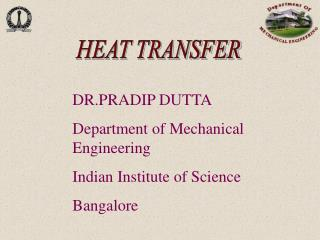DownloadDownload PresentationHEAT TRANSFER

# HEAT TRANSFER

Download Presentation## HEAT TRANSFER

- - - - - - - - - - - - - - - - - - - - - - - - - - - E N D - - - - - - - - - - - - - - - - - - - - - - - - - - -
##### Presentation Transcript

1. HEAT TRANSFER DR.PRADIP DUTTA Department of Mechanical Engineering Indian Institute of Science Bangalore

2. Heat transfer Thermodynamics complementary What is Heat Transfer? “Energy in transit due to temperature difference.” Thermodynamics tells us: • How much heat is transferred (Q) • How much work is done (W) • Final state of the system Heat transfer tells us: • How (with what modes) Q is transferred • At what rate Q is transferred • Temperature distribution inside the body

3. MODES: • Conduction - needs matter - molecular phenomenon (diffusion process) - without bulk motion of matter • Convection - heat carried away by bulk motion of fluid - needs fluid matter • Radiation - does not needs matter - transmission of energy by electromagnetic waves

4. APPLICATIONS OF HEAT TRANSFER • Energy production and conversion - steam power plant, solar energy conversion etc. • Refrigeration and air-conditioning • Domestic applications - ovens, stoves, toaster • Cooling of electronic equipment • Manufacturing / materials processing - welding,casting, soldering, laser machining • Automobiles / aircraft design • Nature (weather, climate etc)

5. T1>T2 T2 T1 .. . . . . . . . . . . . . . . . . . . . . . . . . . . . . . . . . . . . . . . .. . . . . . . . . . . . . . . . . ………….. . .. q’’ (Needs medium, Temperature gradient) CONDUCTION . . . . . . . . . . . . . . . . . . . . . . . . . . . . . solid or stationary fluid RATE: q(W) or (J/s) (heat flow per unit time)

6. x A T1 q T2 k Conduction (contd…) • Rate equations (1D conduction): • Differential Form • q = - k A dT/dx, W • k = Thermal Conductivity, W/mK • A = Cross-sectional Area, m2 • T = Temperature, K or oC • x = Heat flow path, m • Difference Form • q = k A (T1 - T2) / (x1 - x2) Heat flux: q” = q / A = - kdT/dx (W/m2) (negative sign denotes heat transfer in the direction of decreasing temperature)

7. Conduction (contd…) • Example: The wall of an industrial furnace is constructed from 0.2 m thick fireclay brick having a thermal conductivity of 2.0 W/mK. Measurements made during steady state operation reveal temperatures of 1500 and 1250 K at the inner and outer surfaces, respectively. What is the rate of heat loss through a wall which is 0.5 m by 4 m on a side ?

8. Ts>T∞ moving fluid T∞ q” Ts CONVECTION • Energy transferred by diffusion + bulk motion of fluid

9. Rate equation (convection) U  T U  y y u(y) q” T(y) Ts Heat transfer rate q = hA( Ts-T ) W Heat flux q” = h( Ts-T ) W / m2 h=heat transfer co-efficient (W /m2K) (not a property) depends on geometry ,nature of flow, thermodynamics properties etc.

10. Free or natural convection (induced by buoyancy forces) May occur with phase change (boiling, condensation) Convection Forced convection (induced by external means) Convection (contd…)

11. Convection (contd…) Typical values of h (W/m2K) Free convection gases: 2 - 25 liquid: 50 - 100 Forced convection gases: 25 - 250 liquid: 50 - 20,000 Boiling/Condensation 2500 -100,000

12. RATE: q(W) or (J/s) Heat flow per unit time. RADIATION T1 q1” q2” T2 Flux : q” (W/m2)

13. Rate equations (Radiation) RADIATION: Heat Transfer by electro-magnetic waves or photons( no medium required. ) Emissive power of a surface (energy released per unit area): E=Ts4 (W/ m2) = emissivity (property)……… =Stefan-Boltzmann constant

14. Ts u r q”r a d. q”co n v. Ts Area = A Rate equations (Contd….) Radiation exchange between a large surface and surrounding Q”r a d = (Ts4-Tsur4) W/ m2

15. Radiation (contd…) • Example:An uninsulated steam pipe passes through a room in which the air and walls are at 25°C. The outside diameter of pipe is 80 mm, and its surface temperature and emissivity are 180°C and 0.85, respectively. If the free convection coefficient from the surface to the air is 6 W/m2K, what is the rate of heat loss from the surface per unit length of pipe ?